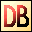DelphiBasics
 RepeatKeyword Repeat statements until a ternmination condition is met
 keyword Repeat(Repeat   Statement1;   {Statement2;   ...}  Until Expression
Description
The Repeat keyword starts a control loop that is always executed at least once, and which terminates when the Expression is satisfied (returns True).

There is no need for Begin or End markers - the Repeat and Until keywords serve that purpose.

It is used when it is important that the statements are at least executed once.
Notes
The last Statement need not have a ; terminator - it is entirely optional.
Related commands
 Begin Keyword that starts a statement block Boolean Allows just True and False values Do Defines the start of some controlled action End Keyword that terminates statement blocks For Starts a loop that executes a finite number of times Until Ends a Repeat control loop While Repeat statements whilst a continuation condition is metDownload this web site as a Windows program.

 Example code : Display integer squares until we reach or exceed 100 var   num, sqrNum : Integer; begin   num := 1;   sqrNum := num * num;   // Display squares of integers until we reach 100 in value   Repeat     // Show the square of num     ShowMessage(IntToStr(num)+' squared = '+IntToStr(sqrNum));     // Increment the number     Inc(num);     // Square the number     sqrNum := num * num;   until sqrNum > 100; end; Show full unit code 1 squared = 1   2 squared = 4   3 squared = 9   4 squared = 16   5 squared = 25   6 squared = 36   7 squared = 49   8 squared = 64   9 squared = 81   10 squared = 100